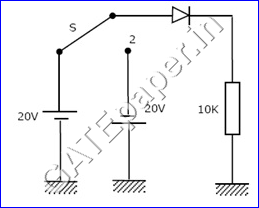## Search this site

### GATE 1991 Electronic Devices (EDC) Video solutions

1.       A silicon sample is uniformly doped with 1016 phosphorous atoms/cm3 and 2 X 1016 boron atoms/cm3. If all the dopants are fully ionized, the material is ………..
2.       An n-type silicon sample, having electron mobility µn twice the hole mobility µp, is subjected to a steady illumination such that the electron concentration doubles from its thermal equilibrium value, as a result, the conductivity of the sample increases by a factor of ………..
3.       The small signal capacitance of an abrupt P+N junction is 1 nF/cm2 at zero bias. If the built in voltage is 1 volt, the capacitance at a reverse bias voltage of 99 volts is equal to ……….
4.       Referring to the circuit shown, the switch is in position 1 initially and steady state conditions exist from time t=0 to t=t0. The switch is suddenly thrown into position 2. The current I flowing through the 10K resistor as function of time from t=0 is  ………. (Give the sketch showing the magnitudes of the current at t=0, t=t0 and t=)5.       Discrete transistors T1 and T2 having maximum collector current rating of 0.75 Amp are connected in parallel as shown in figure. This combination is treated s a single transistor to carry a total current of 1 Amp, when biased with self bias circuit. When the circuit is switched ON, T1 draws 0.55 Amp and T2 draws 0.45 Amp.  If the supply is kept on continuously, ultimately it is very likely that………….6.       The built in potential of the gate junction of an n-channel JFET is 0.5 volts. The drain current saturated at VDS = 5 volts when VGS = 0 volts. The pinch off voltage is …………..

7.       In the figure shown, all transistors are identical and have a high value of beta (β). The voltage VDC is equal to ……………8.       In the figure shown, the input Vi is a 100 Hz triangular wave having a peak to peak amplitude of 2 volts and an average value of zero volts. Given that the diode is ideal, the average value of the output voltage Vo is ……………..
9.       In the figure shown, the n-channel MOSFETs are identical and their current voltage characteristics are given by the following expressions.

Find the current IDC as shown

10.       The current in a forward biased P+N junction shown in figure (a) is entirely due to diffusion of holes from x = 0 to x = L. The injected hole concentration distribution in the n-region is linear as shown in figure (b), with P(0) = 1022 per cm3 and L = 10-3 cm. Determine

a.       The current density in the diode, assuming that the diffusion coefficient of holes is 12 cm2/sec.
b.      The velocity of holes in the n-region at x = 0.

11.        It is required to use a JFET of figure as linear resistor. The parameters of the JFET are as follows. W = 100 µm, L = 10 µm and a = 2.5 µm.  The doping in the n-layer is ND = 1016 cm-3 and the electron mobility is 1500 cm2/V-sec. the depletion layer width of each junction due to the built in potential is 0.25 µm. The two P+ gate regions are connected together externally. The resistances of the regions outside the gate are negligible. Determine the minimum value of the linear resistor which can be realized using this JFET without forward biasing the gate junctions.